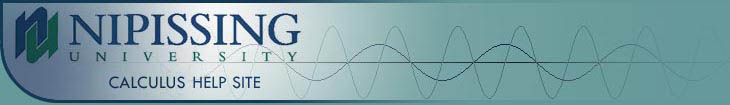TUTORIALS
TUTORIALS HOME

GENERAL MATH
NUMBER SETS
ABSOLUTE VALUE & INEQUALITIES
SETS & INTERVALS
FRACTIONS
POLYNOMIALS
LINEAR EQUATIONS
GEOMETRY
FINITE SERIES
TRIGONOMETRY
EXPONENTS
LOGARITHMS
INDUCTION

CALCULUS
LIMITS
DERIVATIVES
RELATED RATES & OPTIMIZATION
CURVE SKETCHING
INTEGRALS
AREA & VOLUME
INVERSE FUNCTIONS

MAIN
HOME
TESTS
TUTORIALS
SAMPLE PROBLEMS
COMMON MISTAKES
STUDY TIPS
GLOSSARY
CALCULUS APPLICATIONS
MATH HUMOUR

### CURVE SKETCHING TUTORIAL

Before continuing with the curve sketching tutorial, it's recommended that you review the maximum and minimum values section of the related rates and optimization tutorial at the link below.

Maximum and Minimum Values

Curve sketching is another practical application of differential calculus. We can make a fairly accurate sketch of any function using the concepts covered in this tutorial.

# Increasing and Decreasing Functions

The derivative of a function can tell us where the function is increasing and where it is decreasing. If

a) f'(x) > 0 on an interval I, the function is increasing on I.

b) f'(x) < 0 on an interval I, the function is decreasing on I.

The intervals of increase and decrease will occur between points where f'(x) = 0 or f'(x) is undefined. However, these points are not necessarily critical numbers because we include x even if it is not in the domain of f. We simply want to find the intervals of increase and decrease around x, even if the function is not defined at that point.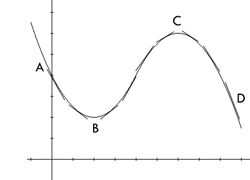The graph to the right illustrates this theorem. From A to B, the slope of the tangent lines are all negative, so the derivative, f'(x) is negative from A to B. The theorem above states that the function is decreasing from A to B. The graph shows that the values of the function are decreasing between A and B. Similarly, the function is also decreasing between C and D. From B to C however, the slopes of the tangent lines are positive. Therefore, the derivative is positive from B to C. The graph shows that the values of the function are increasing between B and C.

# The First Derivative Test

Let c be a critical number of a continuous function f. If

a) f' changes from positive to negative at c, there is a local maximum at c.

b) f' changes from negative to positive at c, there is a local minimum at c.

c) f' does not change sign at c, (that is, the derivative is positive before and after c or negative before and after c) there is no maximum or minimum at c.

The graphs below illustrate the first derivative test.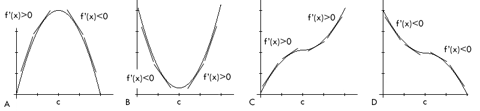# Concavity and Points of Inflection

A graph is called concave upward (CU) on an interval I, if the graph of the function lies above all of the tangent lines on I. A graph is called concave downward (CD) on an interval I, if the graph of the function lies below all of the tangent lines on I.

The second derivative of a function can tell us whether a function is concave upward or concave downward. If

a) f''(x) > 0 for all x in an interval I, the graph is concave upward on I.

b) f''(x) < 0 for all x in an interval I, the graph is concave downward on I.

The intervals of concavity will occur between points where f''(x) = 0 or f''(x) is undefined. We test the concavity around these points even if they are not included in the domain of f.

The graphs below illustrate the different forms of concavity. Remember that there are two ways in which a graph can be concave upward or concave downward. Graphs A and C illustrate the types of concavity when the function is increasing on the interval, while graphs B and D illustrate concavity when the function is decreasing on the interval. These 4 graphs cover every different form of concavity.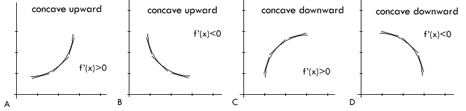A point P on a curve is called a point of inflection if the function is continuous at that point and either

a) the function changes from CU to CD at P
b) the function changes from CD to CU at P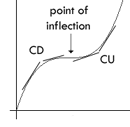Points of inflection may occur at points where f''(x) = 0 or f''(x) is undefined, where x is in the domain of f. We must test the concavity around these points to determine whether they are points of inflection.

The graph to the right illustrates a curve with a point of inflection.

# The Second Derivative Test

Let f be a continuous function near c. If

a) f'(c) = 0 and f''(c) > 0, then f has a local minmum at c.
b) f'(c) = 0 and f''(c) < 0, then f has a local maximum at c.

The graphs containing local maximums and minimums in the "Increasing and Decreasing Functions" and "The First Derivative Test" sections above illustrate the second derivative test. When a graph has a local minimum, the function is concave upward (and thus lies above the tangent lines) at the minimum. Similarly, the function is concave downward at a local maximum.

# Examples

1 | Use the graph to find the following
2 | For the function f, find the following

# Asymptotes

Before continuing with asymptotes, it is recommended that you review the vertical asymptote and infinite limits section of the limits tutorial at the link below.

Vertical Asymptotes and Infinite Limits

In order to properly sketch a curve, we need to determine how the curve behaves as x approaches positive and negative infinity. We must find the limit of the function as x approaches infinity.

For a function f defined on (a, ¥),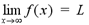means that the values of f(x) approach the value L when x is taken to be sufficiently large.

For a function f defined on (-¥, a),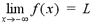means that the values of f(x) approach the value L when x is taken to be sufficiently large, negatively.

The line y = L is called a horizontal asymptote of y = f(x) if either,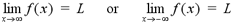In order to find the horizontal asymptotes of a function, we use the following theorem. If n is a positive number, then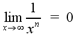If n is a positive, rational number such that xn is defined for all x, then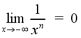Functions do not always approach a value as x approaches positive or negative infinity. Often there is no horizontal asymptote and the functions have infinite limits at infinity. For example, the function f(x) = x2 approaches infinity when x is taken to be sufficiently large, positively or negatively.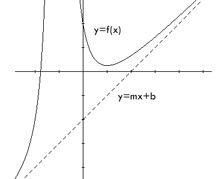# Slant Asymptotes

Some curves may have an asymptote that is neither vertical nor horizontal. These curves approach a line as x approaches positive or negative infinity. This line is called the slant asymptote of the function. The graph to the right illustrates the concept of slant asymptotes.

If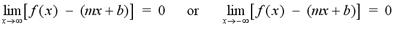then the function f(x) has a slant asymptote of y = mx + b.

Rational functions will have a slant asymptote when the degree of the numerator is one more than the degree of the denominator. To find the equation of the slant asymptote, we divide the numberator by the denominator using long division. The quotient will be the equation of the slant asymptote. The remainder is the quantity f(x) - (mx + b). We must show that the remainder approaches 0, as x approaches positive or negative infinity. The example below will give you a better idea of how to find the slant asymptote of a function.

# Examples

3 | Evaluate the limit
4 | Find the vertical and horizontal asymptotes of the function
5 | Find the equation of the slant asymptote of the function

# Curve Sketching

The following steps are helpful when sketching curves. These are general guidelines for all curves, so each step may not always apply to all functions.

a) Domain: Find the domain of the function. This will be useful when finding vertical asymptotes and determining critical numbers.

b) Intercepts: Find the x- and y-intercepts of the function, if possible. To find the x-intercept, we set y = 0 and solve the equation for x. Similarly, we set x = 0 to find the y-intercept.

c) Symmetry: Determine whether the function is an odd function, an even function or neither odd nor even. If f(-x) = f(x) for all x in the domain, then f is even and symmetric about the y-axis. If f(-x) = -f(x) for all x in the domain, then f is odd and symmetric about the origin.

d) Asymptotes: Find the asymptotes of the function using the methods described above. First attempt to find the vertical and horizontal asymptotes of the function. IF necessary, find the slant asymptote.

e) Intervals of Increase and Decrease: Using the methods described above, determine where f'(x) is positive and negative to find the intervals where the function is increasing and decreasing.

f) Local Maximum/Minimum : Find the critical numbers of the function. Remember that the number c in the domain is a critical number if f'(c) = 0 or f'(c) does not exist. Use the first derivative test to find the local maximums and minimums of the function.

g) Concavity and Points of Inflection : We must determine when f''(x) is positive and negative to find the intervals where the function is concave upward and concave downward. Inflection points occur whenever the curve changes in concavity.

h) Sketch : Using the information obtained from steps A to G, we can sketch the curve. First, we draw dashed lines for the asymptotes of the function. Then plot the x- and y-intercepts, maximum and minimum points and points of inflection on the graph. Sketch the curve between the points, using the intervals of increase and decrease and intervals of concavity. Be sure that the graph behaves correctly when approaching asymptotes.

The examples below will give you a better idea of how to follow these steps to sketch the graph of a function.

# Examples

6 | Sketch the graph of the function ( Steps A to D )
| Sketch the graph of the function ( Steps E to H )
7 | Sketch the graph of the function ( Steps A to D )
| Sketch the graph of the function ( Steps E to H )

For more practice with the concepts covered in this tutorial, visit the Curve Sketching Problems page at the link below. The solutions to the problems will be posted after these chapters are covered in your calculus course.

To test your knowledge of curve sketching problems, try taking the general curve sketching test on the iLrn website or the advanced curve sketching test at the link below.

Curve Sketching Problems
General Curve Sketching Test on iLrn
Advanced Curve Sketching Test

|Top of Page |

COURSE HOMEPAGES
MATH 1036
MATH 1037

FACULTY HOMEPAGES
Alex Karassev
Ted Chase